Mathematical and Physical Journal
for High Schools
Issued by the MATFUND Foundation
 Already signed up? New to KöMaL?

# Exercises and problems in PhysicsJanuary 2004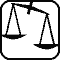## Experimental problem

M. 248. It is said that hot water placed in the deep-freezer freezes sooner than cold water. Check this statement with water samples of the same amount (relatively small amounts) and of different temperatures.

(6 points)## Theoretical problems

It is allowed to send solutions for any number of problems, but final scores of students of grades 9-12 are computed from the 5 best score in each month. Final scores of students of grades 1-8 are computed from the 3 best scores in each month.

P. 3672. Two rockets are launched at the same time towards each other from their launch pads 60 km away from each other. The speeds of the rockets are 116 km/h and 184 km/h, respectively. How far are they from each other one and a half minutes before their collision?

(3 points)

P. 3673. In a water tank the water level is at height H. There is a little hole in the side of the tank at height h from its bottom, from where the water squirts out horizontally. At what value of h does the jet of water hit the horizontal ground furthest, and what is this distance?

(4 points)

P. 3674. Can three celestial bodies move uniformly in circular orbits so that they keep constant distances from each other in the meantime?

(5 points)

P. 3675. Two thin solid disks of mass m and radius R can rotate at the ends of an axle of negligible mass. This system is placed on a horizontal table and is rotated around its center so that the disks roll clearly on the table. The centers of both disks move at a speed of v0 on a circular track of radius r. What is the entire kinetic energy of the system?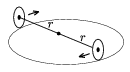(5 points)

P. 3676. Two point sound sources emit sounds of the same frequency in the same phase. The distance of the sound sources is AB=40 m. If the frequency is changed between frequencies 670 Hz and 690 Hz, intensification can be experienced at point C on line AB located 5 meters from A. a) At what frequency does this intensification happen? b) What can we experience at point D which is located on a line perpendicular to AB and starting in C at a 12 m distance from line AB? (The speed of sound is 340 m/s).

(4 points)

P. 3677. An ideal gas is going through a process that is shown in the figure. During the process its temperature rises then decreases. What is the volume of the gas when it reaches its maximum temperature?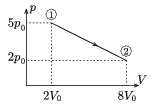(4 points)

P. 3678. Two long parallel conductor cables at a 0.1 m distance of each other are electrically charged. The charge on one of them is 10-7 C per meter and 2.10-7 C per meter on the other. What force is exerted on a 0.5 m long piece of one of the cables?

(4 points)

P. 3679. Two long straight wires are connected to a thin-walled spherical metal shell of radius R directed towards the centre of the sphere. The wires are perpendicular to each other. Current I is lead to the sphere in one of the wires and in the other wire it is lead away from the sphere. What is the magnitude of the magnetic induction vector in the centre of the spherical shell and what is its direction?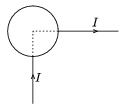(5 points)

P. 3680. On a horizontal surface there are two coarse-surfaced solid balls of the same mass and radius rolling slip-free towards each other along the same line with velocities v0=4.20 m/s. At a given moment the distance of the balls is d=12.60 m. How long after this moment are they again in a distance d from each other, if their collision is instantaneous and absolutely elastic? The friction coefficient is 0.16 everywhere and the rolling-friction and the air drag are negligible.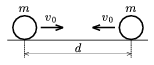(5 points)

P. 3681. To detect the electron neutrinos ($\displaystyle \nu$e) arriving from the Sun physicists use materials containing chlorine: $\displaystyle \nu_{\rm e}+{\null}^{37}{\rm Cl}~\to~{\null}^{37}{\rm Ar}+{\rm e}^-$. Considering the conservation of energy and momentum, determine what is the energy content of such a neutrino at the least. (Data: the mass of the chlorine nucleus is 39.945 60 ATE, the mass of the argon nucleus is 36.946 47 ATE.)

(5 points)